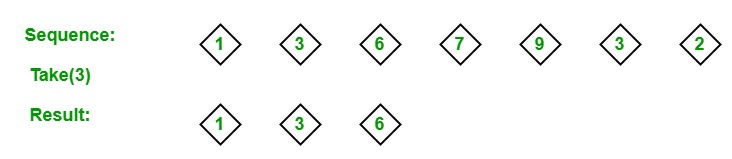# LINQ | Partition Operator | Take

• Last Updated : 28 May, 2019

In LINQ, partition operators are used for separating the given sequence into two portions without sorting the elements and return one of the portions. The Standard Query Operators supports 4 different types of partition operators:

1. Skip
2. SkipWhile
3. Take
4. TakeWhile

#### Take Operator

The Take operator is used to return a specified number of adjacent elements from the start of the sequences. Or in other words, we can say that it returns the specified number of items starting from the first item. As shown in the below example:Important Points:

• It does not support query syntax in C# and VB.Net languages. But you can use the Take method to query variable or you can wrap your query in brackets and then the call Take method. As shown in the Example 2.
• It support method syntax in both C# and VB.Net languages.
• It present in both the Queryable and Enumerable class.
• It is implemented by using deferred execution.
• It will throw an ArgumentNullException if the source is null.

Example 1:

 `// C# program to illustrate the``// concept of Taking operator``using` `System;``using` `System.Linq;`` ` `class` `GFG {`` ` `    ``// Main Method``    ``static` `public` `void` `Main()``    ``{`` ` `        ``// Data source``        ``int``[] sequence = {45, 67, 89,``                     ``13, 56, 76, 67};`` ` `        ``// Query which picks starting 5 ordered``        ``// elements from the given sequence``        ``// Using Take method``        ``var` `result = sequence.OrderBy(s => s).Take(5);`` ` `        ``Console.WriteLine(``"New Sequence: "``);`` ` `        ``// Display new sequence``        ``foreach``(``var` `val ``in` `result)``        ``{``            ``Console.WriteLine(val);``        ``}``    ``}``}`

Output:

```New Sequence:
13
45
56
67
67
```

Example 2:

 `// C# program to find the names``// of the first 4 employees``using` `System;``using` `System.Linq;``using` `System.Collections.Generic;`` ` `// Employee details``public` `class` `Employee {`` ` `    ``public` `int` `emp_id``    ``{``        ``get``;``        ``set``;``    ``}``    ``public` `string` `emp_name``    ``{``        ``get``;``        ``set``;``    ``}``    ``public` `string` `emp_gender``    ``{``        ``get``;``        ``set``;``    ``}``    ``public` `string` `emp_hire_date``    ``{``        ``get``;``        ``set``;``    ``}``    ``public` `int` `emp_salary``    ``{``        ``get``;``        ``set``;``    ``}``}`` ` `public` `class` `GFG {`` ` `    ``// Main method``    ``static` `public` `void` `Main()``    ``{`` ` `        ``List emp = ``new` `List() {`` ` `            ``new` `Employee() {emp_id = 209, emp_name = ``"Anjita"``, emp_gender = ``"Female"``,``                                    ``emp_hire_date = ``"12/3/2017"``, emp_salary = 20000},`` ` `            ``new` `Employee() {emp_id = 210, emp_name = ``"Soniya"``, emp_gender = ``"Female"``,``                                    ``emp_hire_date = ``"22/4/2018"``, emp_salary = 30000},`` ` `            ``new` `Employee() {emp_id = 211, emp_name = ``"Rohit"``, emp_gender = ``"Male"``,``                                  ``emp_hire_date = ``"3/5/2016"``, emp_salary = 40000},`` ` `            ``new` `Employee() {emp_id = 212, emp_name = ``"Anu"``, emp_gender = ``"Female"``,``                                  ``emp_hire_date = ``"4/8/2017"``, emp_salary = 80000},`` ` `            ``new` `Employee() {emp_id = 213, emp_name = ``"Anil"``, emp_gender = ``"Male"``,``                                ``emp_hire_date = ``"12/1/2016"``, emp_salary = 60000},`` ` `            ``new` `Employee() {emp_id = 214, emp_name = ``"Anju"``, emp_gender = ``"Female"``,``                                  ``emp_hire_date = ``"17/6/2015"``, emp_salary = 50000},``        ``};`` ` `        ``// Query to find the names ``        ``// of the first 4 employees``        ``// Using Take method``        ``var` `res = (``from` `e ``in` `emp``                   ``select` `e.emp_name)``                   ``.Take(4);`` ` `        ``foreach``(``var` `val ``in` `res)``        ``{``            ``Console.WriteLine(``"Employee Name: {0}"``, val);``        ``}``    ``}``}`

Output:

```Employee Name: Anjita
Employee Name: Soniya
Employee Name: Rohit
Employee Name: Anu
```

My Personal Notes arrow_drop_up